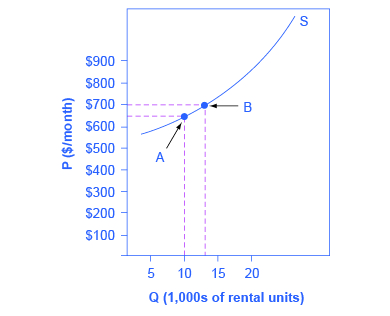Price Elasticity of Supply

Learning Objectives

• Calculate the price elasticity of supply

Calculating the Price Elasticity of Supply

The price elasticity of supply measures how much quantity supplied changes in response to a change in the price. The calculations and interpretations are analogous to those we explained above for the price elasticity of demand. The only difference is we are looking at how producers respond to a change in the price instead of how consumers respond.

Price elasticity of supply is the percentage change in the quantity of a good or service supplied divided by the percentage change in the price. Since this elasticity is measured along the supply curve, the law of supply holds, and thus price elasticities of supply are always positive numbers. We describe supply elasticities as elastic, unitary elastic and inelastic, depending on whether the measured elasticity is greater than, equal to, or less than one.

Exercise: Elasticity of Supply from Point A to Point B

Assume that an apartment rents for $650 per month and at that price 10,000 units are offered for rent, as shown in Figure 2, below. When the price increases to$700 per month, 13,000 units are offered for rent. By what percentage does apartment supply increase? What is the price sensitivity?Figure 2. Price Elasticity of Supply. The price elasticity of supply is calculated as the percentage change in quantity divided by the percentage change in price.

Step 1. We know that

$\displaystyle\text{Price Elasticity of Supply}=\frac{\text{percent change in quantity}}{\text{percent change in price}}$

Step 2. From the midpoint method we know that

$\displaystyle\text{percent change in quantity}=\frac{Q_2-Q_1}{(Q_2+Q_1)\div{2}}\times{100}$

$\displaystyle\text{percent change in price}=\frac{P_2-P_1}{(P_2+P_1)\div{2}}\times{100}$

Step 3. We can use the values provided in the figure in each equation:

$\displaystyle\text{percent change in quantity}=\frac{13,000-10,000}{(13,000+10,000)\div{2}}\times{100}=\frac{3,000}{11,500}\times{100}=26.1$

$\displaystyle\text{percent change in price}=\frac{700-650}{(700+650)\div{2}}\times{100}=\frac{50}{675}\times{100}=7.4$

Step 4. Then, those values can be used to determine the price elasticity of demand:

$\displaystyle\text{Price Elasticity of Supply}=\frac{26.1\text{ percent}}{7.4\text{ percent}}=3.53$

Again, as with the elasticity of demand, the elasticity of supply is not followed by any units. Elasticity is a ratio of one percentage change to another percentage change—nothing more—and is read as an absolute value. In this case, a 1% rise in price causes an increase in quantity supplied of 3.5%. Since 3.5 is greater than 1, this means that the percentage change in quantity supplied will be greater than a 1% price change. If you’re starting to wonder if the concept of slope fits into this calculation, read on for clarification.

Watch It

Watch this video to see a real-world application of price elasticity.

Try It

These questions allow you to get as much practice as you need, as you can click the link at the top of the first question (“Try another version of these questions”) to get a new set of questions. Practice until you feel comfortable doing the questions.

Glossary

elastic supply:
supply responds more than proportionately to a change in price; i.e. the percent change in quantity supplied is greater than percent change in price
inelastic supply:
supply responds less than proportionately to a change in price; i.e. the percent change in quantity supplied is less than percent change in price
price elasticity of supply:
percentage change in the quantity supplied divided by the percentage change in price
unitary elastic supply:
supply responds exactly proportionately to a change in price; i.e. the percent change in quantity supplied is equal to the percent change in price